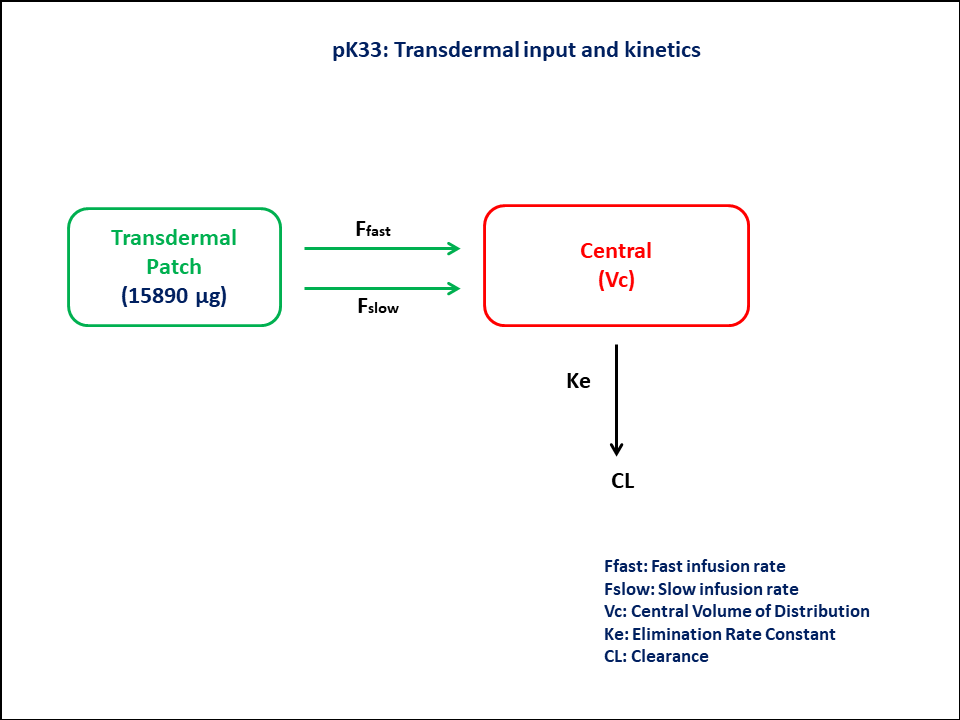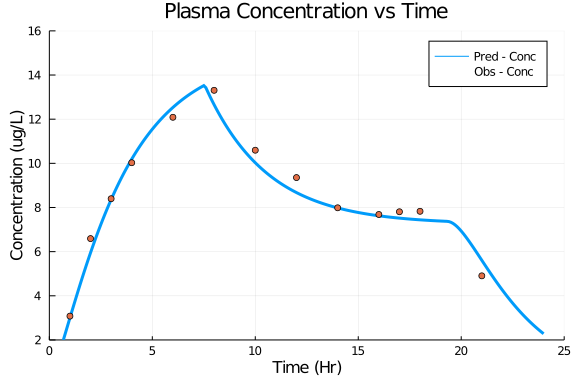# Exercise 33 - Transdermal input and kinetics

### Background

• Structural model - One compartment linear elimination with zero-order input

• Route of administration - Transdermal

• Dosage Regimen - 15,890 μg per patch. The patch was applied for 16 hours for 5 consecutive days

• Number of Subjects - 1### Learning Outcome

To understand the kinetics of a given drug using Transdermal input following 2 different input rates

### Objectives

To build one compartment model with zero-order input and to understand its function using transdermal delivery system.

### Libraries

Call the "necessary" libraries to get started.

using Pumas
using Plots
using CSV
using StatsPlots
using Random


### Model

To build one compartment model with zero-order input following transdermal drug administration

pk_33         = @model begin
@param begin
tvcl      ∈ RealDomain(lower=0)
tvvc      ∈ RealDomain(lower=0)
tvdslow   ∈ RealDomain(lower=0)
tvtfast   ∈ RealDomain(lower=0)
tvtslow   ∈ RealDomain(lower=0)
Ω         ∈ PDiagDomain(5)
σ_prop    ∈ RealDomain(lower=0)
end

@random begin
η         ~ MvNormal(Ω)
end

@pre begin
Cl        = tvcl * exp(η)
Vc        = tvvc * exp(η)
Dose_slow = (tvdslow/15890) * exp(η)
Tfast     = tvtfast * exp(η)
Tslow     = tvtslow * exp(η)
duration  = (Central=Tfast, Dslow=Tslow)
bioav     = (Central=1-Dose_slow, Dslow=Dose_slow)
end

@dynamics begin
Dslow'    = -Dslow
Central'  =  Dslow -(Cl/Vc)*Central
end

@derived begin
cp        = @. Central/Vc
dv        ~ @. Normal(cp, sqrt(cp^2*σ_prop))
end
end

PumasModel
Parameters: tvcl, tvvc, tvdslow, tvtfast, tvtslow, Ω, σ_prop
Random effects: η
Covariates:
Dynamical variables: Dslow, Central
Derived: cp, dv
Observed: cp, dv


### Parameters

Parameters provided for simulation are as below. tv represents the typical value for parameters.

• CL - Clearance (L/hr),

• Vc - Volume of Central Compartment (L)

• Dslow - Dose of slow infusion (μg)

• Tfast - Duration of fast release (hr)

• Tslow - Duration of slow release (hr)

• Ω - Between Subject Variability

• σ - Residual error

param = ( tvcl    = 79.8725,
tvvc    = 239.94,
tvdslow = 11184.3,
tvtfast = 7.54449,
tvtslow = 19.3211,
Ω       = Diagonal([0.0,0.0,0.0,0.0,0.0]),
σ_prop  = 0.005)

(tvcl = 79.8725, tvvc = 239.94, tvdslow = 11184.3, tvtfast = 7.54449, tvtsl
ow = 19.3211, Ω = [0.0 0.0 … 0.0 0.0; 0.0 0.0 … 0.0 0.0; … ; 0.0 0.0 … 0.0
0.0; 0.0 0.0 … 0.0 0.0], σ_prop = 0.005)


### DosageRegimen

• 15,890 μg per patch.

• The patch is applied for 16 hours, for 5 consecutive days

• The patch releases the drug at two different rate processes, fast and slow simultaneously over a period of 6 and 18 hours respectively.

ev1  = DosageRegimen([15890,15890], time=0, cmt = [1,2], rate=[-2,-2])
sub1 = Subject(id=1, events=ev1)

Subject
ID: 1
Events: 4


### Simulation

Simulate the plasma concentration

Random.seed!(123)
sim_sub1 = simobs(pk_33, sub1, param, obstimes=0:0.1:24)
df1      = DataFrame(sim_sub1)


### DataFrame & Plot

Create a dataframe of the simulated data and to plot it.

df1_dv = filter(x -> x.time in [0,0.5,1,2,3,4,6,8,10,12,14,16,17,18,21,23.37], df1)

@df df1 plot(:time, :cp,
title="Plasma Concentration vs Time", label= "Pred - Conc",
xlabel= "Time (Hr)", ylabel="Concentration (ug/L)", linewidth=3,
xlims=(0,25), xticks=[0,5,10,15,20,25], yticks=[2,4,6,8,10,12,14,16], ylims=(2,16))
@df df1_dv scatter!(:time, :dv, label="Obs - Conc")